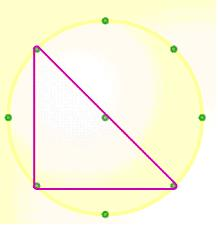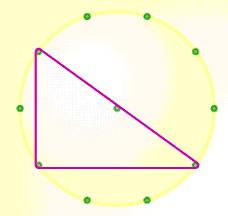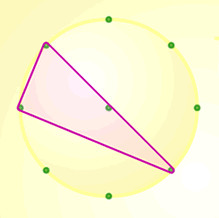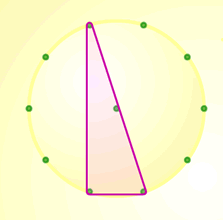#### You may also like### Coins on a Plate

Points A, B and C are the centres of three circles, each one of which touches the other two. Prove that the perimeter of the triangle ABC is equal to the diameter of the largest circle.### Not So Little X

Two circles are enclosed by a rectangle 12 units by x units. The distance between the centres of the two circles is x/3 units. How big is x?This gives a short summary of the properties and theorems of cyclic quadrilaterals and links to some practical examples to be found elsewhere on the site.

# Right Angles

##### Age 11 to 14 Challenge Level:

Samantha and Shummus both realised that in order to create a triangle with a right angle, the band had to go through the centre of the circle. Shummus writes:

I noticed that the bands had to be started in the centre.

Xianglong Ni notes that:

If we have 9 points on the circle then you can't create a right-angle using the points. This is so because a right angle is inscribed in a semicircle; It is facing a diameter. But you can only create a diameter when there is an even amount of points on the circle. If the number of points on the circle is even then yes. If the number is odd then no.

Rachel from Newstead sent us a few diagrams to illustrate examples of right-angled triangles in circles with an even number of points.Indika of Helena Romanes 6th Form College sent us her explanation for why the band must go through the centre of the circle:

The only way that a right angle triangle can be created between 3 points round the edge is when the angle subtended at the centre by two of the points is 180 degrees, this therefore proves that two of the points have to be opposite each other (this means having an equal number of pegs).

This is because the angle subtended at the centre by two points are exactly double the angle subtended at the edge by the same points. This rule will apply to all circles, i.e. there will be a right angled triangle if two pegs are placed opposite each other.

If you haven't met this idea before, you may want to look at another problem from August 2005, Subtended angles

Here are another couple of examples of right-angled triangles using the same eight-point and ten-point circles that Rachel used: